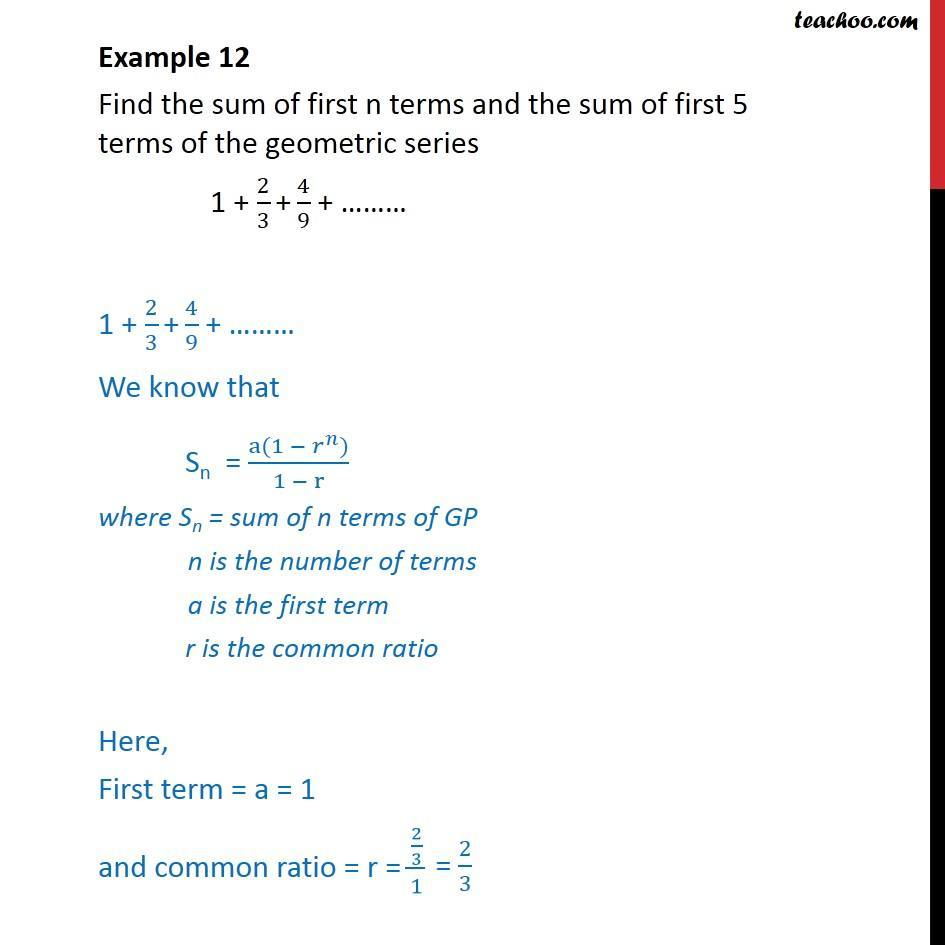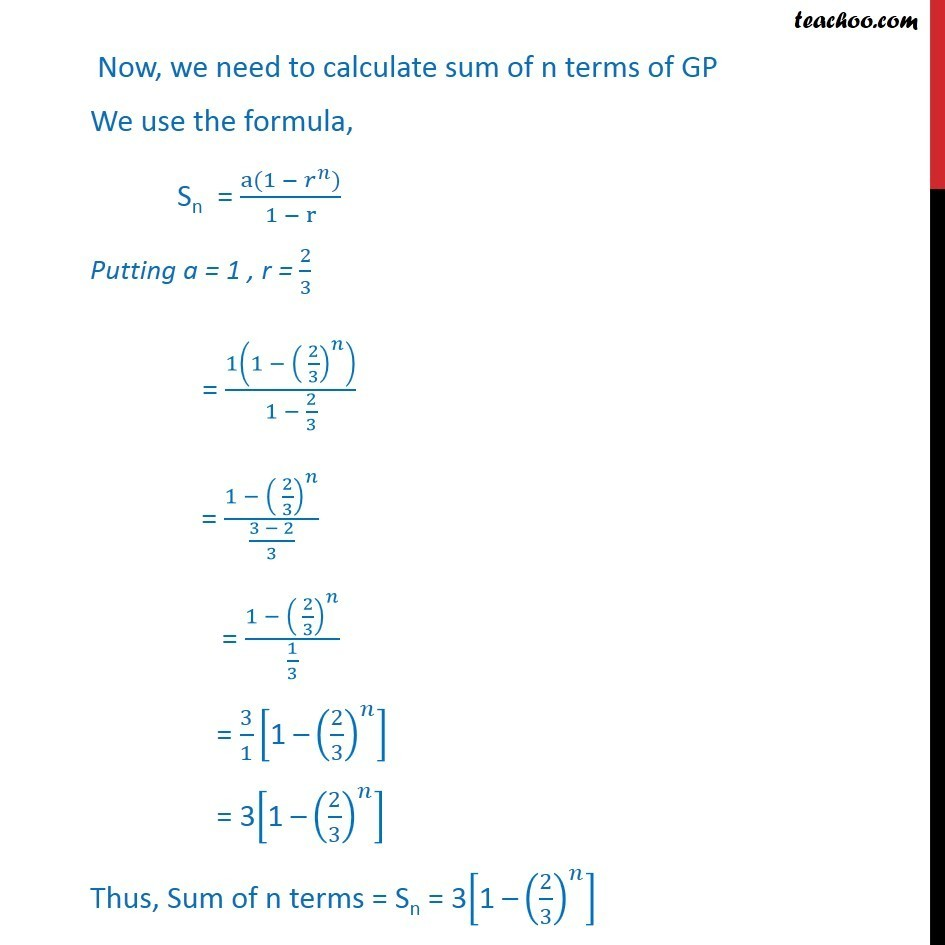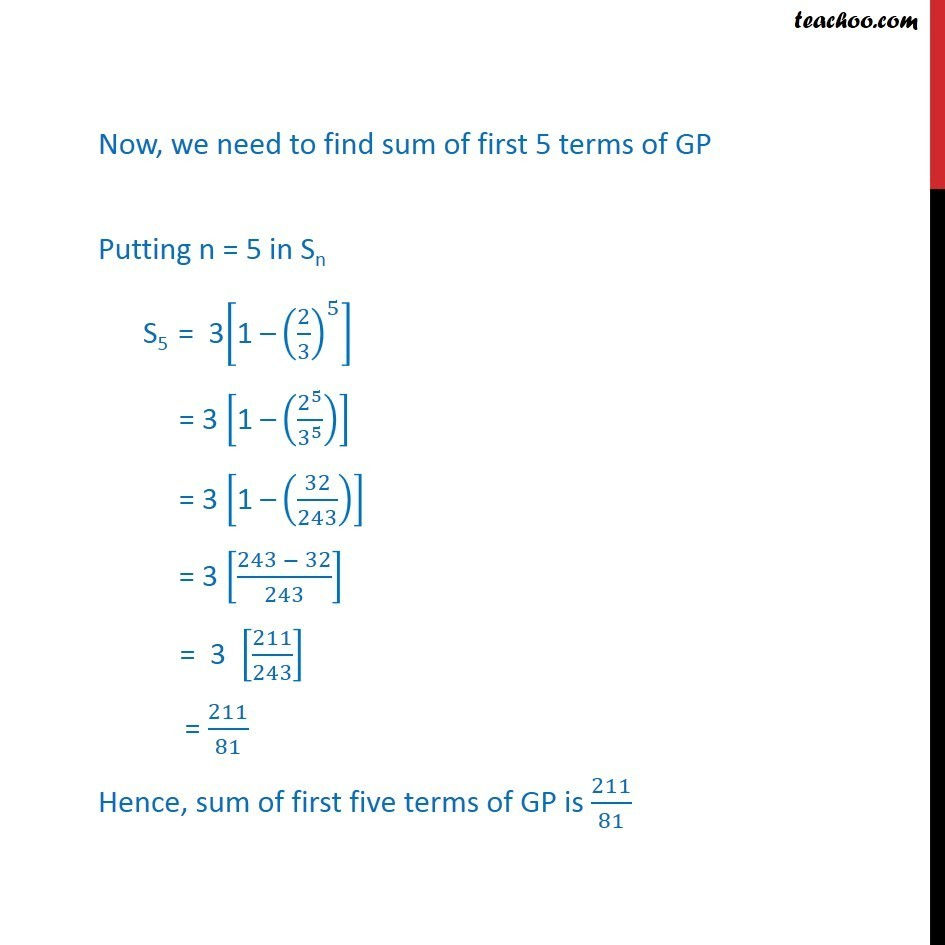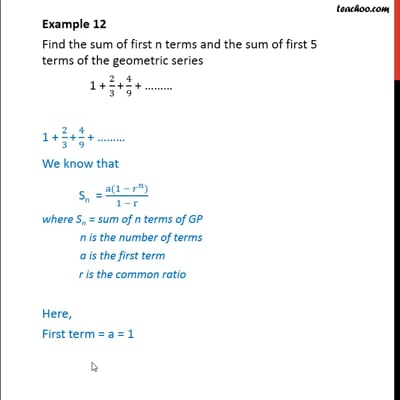Examples

Chapter 9 Class 11 Sequences and Series (Term 1)
Serial order wiseThis video is only available for Teachoo black users

### Transcript

Example 12 Find the sum of first n terms and the sum of first 5 terms of the geometric series 1 + 2/3 "+" 4/9 + ……… 1 + 2/3 "+" 4/9 + ……… We know that Sn = (a(1 − 𝑟^𝑛))/(1 − r) where Sn = sum of n terms of GP n is the number of terms a is the first term r is the common ratio Here, First term = a = 1 and common ratio = r = (2/3)/1 Now, we need to calculate sum of n terms of GP We use the formula, Sn = (a(1 − 𝑟^𝑛))/(1 − r) Putting a = 1 , r = 2/3 = 1(1 − ( 2/3)^𝑛 )/(1 − 2/3) = (1 − ( 2/3)^𝑛)/((3 − 2)/3) = (1 − ( 2/3)^𝑛)/(1/3) = 3/1 ["1 –" (2/3)^𝑛 ] = 3["1 –" (2/3)^𝑛 ] Thus, Sum of n terms = Sn = 3["1 –" (2/3)^𝑛 ] Now, we need to find sum of first 5 terms of GP Putting n = 5 in Sn S5 = 3["1 –" (2/3)^5 ] = 3 ["1 –" (2^5/3^5 )] = 3 ["1 –" (32/243)] = 3 [(243 − 32)/243] = 3 [211/243] = 211/81 Hence, sum of first five terms of GP is 211/81